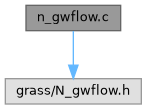GRASS GIS 8 Programmer's Manual  8.2.2dev(2023)-5c00b4e944
n_gwflow.c File Reference
#include <grass/N_gwflow.h>
Include dependency graph for n_gwflow.c:Go to the source code of this file.

## Functions

N_gwflow_data3dN_alloc_gwflow_data3d (int cols, int rows, int depths, int river, int drain)
Alllocate memory for the groundwater calculation data structure in 3 dimensions. More...

void N_free_gwflow_data3d (N_gwflow_data3d *data)
Release the memory of the groundwater flow data structure in three dimensions. More...

N_gwflow_data2dN_alloc_gwflow_data2d (int cols, int rows, int river, int drain)
Alllocate memory for the groundwater calculation data structure in 2 dimensions. More...

void N_free_gwflow_data2d (N_gwflow_data2d *data)
Release the memory of the groundwater flow data structure in two dimensions. More...

N_data_starN_callback_gwflow_3d (void *gwdata, N_geom_data *geom, int col, int row, int depth)
This callback function creates the mass balance of a 7 point star. More...

void N_gwflow_3d_calc_water_budget (N_gwflow_data3d *data, N_geom_data *geom, N_array_3d *budget)
This function computes the water budget of the entire groundwater. More...

N_data_starN_callback_gwflow_2d (void *gwdata, N_geom_data *geom, int col, int row)
This callback function creates the mass balance of a 5 point star. More...

void N_gwflow_2d_calc_water_budget (N_gwflow_data2d *data, N_geom_data *geom, N_array_2d *budget)
This function computes the water budget of the entire groundwater. More...

## ◆ N_alloc_gwflow_data2d()

 N_gwflow_data2d* N_alloc_gwflow_data2d ( int cols, int rows, int river, int drain )

Alllocate memory for the groundwater calculation data structure in 2 dimensions.

The groundwater calculation data structure will be allocated including all appendant 2d arrays. The offset for the 3d arrays is one to establish homogeneous Neumann boundary conditions at the calculation area border. This data structure is used to create a linear equation system based on the computation of groundwater flow in porous media with the finite volume method.

Parameters
 cols int rows int
Returns
N_gwflow_data2d *

Definition at line 148 of file n_gwflow.c.

## ◆ N_alloc_gwflow_data3d()

 N_gwflow_data3d* N_alloc_gwflow_data3d ( int cols, int rows, int depths, int river, int drain )

Alllocate memory for the groundwater calculation data structure in 3 dimensions.

The groundwater calculation data structure will be allocated including all appendant 3d and 2d arrays. The offset for the 3d arrays is one to establish homogeneous Neumann boundary conditions at the calculation area border. This data structure is used to create a linear equation system based on the computation of groundwater flow in porous media with the finite volume method.

Parameters
 cols int rows int depths int
Returns
N_gwflow_data3d *

Definition at line 38 of file n_gwflow.c.

## ◆ N_callback_gwflow_2d()

 N_data_star* N_callback_gwflow_2d ( void * gwdata, N_geom_data * geom, int col, int row )

This callback function creates the mass balance of a 5 point star.

The mass balance is based on the common groundwater flow equation:This equation is discretizised with the finite volume method in two dimensions.

Parameters
 gwdata N_gwflow_data2d * geom N_geom_data * col int row int
Returns
N_data_star *

Definition at line 464 of file n_gwflow.c.

References N, r, and W.

## ◆ N_callback_gwflow_3d()

 N_data_star* N_callback_gwflow_3d ( void * gwdata, N_geom_data * geom, int col, int row, int depth )

This callback function creates the mass balance of a 7 point star.

The mass balance is based on the common groundwater flow equation:This equation is discretizised with the finite volume method in three dimensions.

Parameters
 gwdata N_gwflow_data3d * geom N_geom_data * col int row int depth int
Returns
N_data_star *

Definition at line 263 of file n_gwflow.c.

## ◆ N_free_gwflow_data2d()

 void N_free_gwflow_data2d ( N_gwflow_data2d * data )

Release the memory of the groundwater flow data structure in two dimensions.

Parameters
 data N_gwflow_data2d *
Returns
void

Definition at line 200 of file n_gwflow.c.

## ◆ N_free_gwflow_data3d()

 void N_free_gwflow_data3d ( N_gwflow_data3d * data )

Release the memory of the groundwater flow data structure in three dimensions.

Parameters
 data N_gwflow_data3d *
Returns
void *

Definition at line 92 of file n_gwflow.c.

## ◆ N_gwflow_2d_calc_water_budget()

 void N_gwflow_2d_calc_water_budget ( N_gwflow_data2d * data, N_geom_data * geom, N_array_2d * budget )

This function computes the water budget of the entire groundwater.

The water budget is calculated for each active and dirichlet cell from its surrounding neighbours. This is based on the 5 star mass balance computation of N_callback_gwflow_2d and the gradient of the water heights in the cells. The sum of the water budget of each active/dirichlet cell must be near zero due the effect of numerical inaccuracy of cpu's.

Parameters
 gwdata N_gwflow_data2d * geom N_geom_data * budget N_array_2d
Returns
void

Definition at line 659 of file n_gwflow.c.

## ◆ N_gwflow_3d_calc_water_budget()

 void N_gwflow_3d_calc_water_budget ( N_gwflow_data3d * data, N_geom_data * geom, N_array_3d * budget )

This function computes the water budget of the entire groundwater.

The water budget is calculated for each active and dirichlet cell from its surrounding neighbours. This is based on the 7 star mass balance computation of N_callback_gwflow_3d and the gradient of the water heights in the cells. The sum of the water budget of each active/dirichlet cell must be near zero due the effect of numerical inaccuracy of cpu's.

Parameters
 gwdata N_gwflow_data3d * geom N_geom_data * budget N_array_3d
Returns
void

Definition at line 371 of file n_gwflow.c.